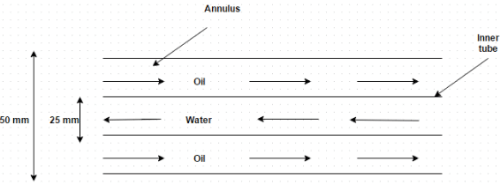# Test: Number of Transfer Units

## 10 Questions MCQ Test Heat Transfer | Test: Number of Transfer Units

Description
Attempt Test: Number of Transfer Units | 10 questions in 10 minutes | Mock test for Chemical Engineering preparation | Free important questions MCQ to study Heat Transfer for Chemical Engineering Exam | Download free PDF with solutions
QUESTION: 1

### Pick the odd one out

Solution:

In surface condenser, the heat transfer occurs between the fluid streams without mixing or physical contact with each other.

QUESTION: 2

### The lubricating oil for a large industrial gas turbine engine is cooled in a counter flow, concentric tube heat exchanger. The cooling water flows through the inner tube ( diameter = 25 mm) with inlet temperature 25 degree celsius and mass flow rate 0.2 kg/s. The oil flows through the annulus (diameter = 50 mm) with mass flow rate 0.125 kg/s and its temperature at entry and exit are 90 degree Celsius and 60 degree Celsius. Find outlet temperature of cooling waterSolution:

mc(th1 – th2) = mcc(tc2 – tc1).

QUESTION: 3

### A cross flow type air heater has an area of 50 cm2. The overall heat transfer coefficient is 100 W/m2 K and heat capacity of both hot and cold stream is 1000 W/m K. The value of NTU is

Solution:

NTU = A U/C MIN = 5.

QUESTION: 4

In a balanced counter flow heat exchanger with mh c= mcc, the NTU is equal to unity. What is the effectiveness of heat exchanger?

Solution:
QUESTION: 5

After expansion from a gas turbine, the hot exhaust gases are used to heat the compressed air from a compressor with the help of a cross flow heat exchanger of 0.8 effectiveness. What is the number of transfer units of the heat exchanger?

Solution:

Effectiveness = NTU/NTU – 1 = 0.5.

QUESTION: 6

E – NTU method is particularly useful in thermal design of heat exchangers when

Solution:

It is useful when outlet temperatures of the fluid streams are not known as a priori.

QUESTION: 7

The engine oil at 150 degree Celsius is cooled to 80 degree Celsius in a parallel flow heat exchanger by water entering at 25 degree Celsius and leaving at 60 degree Celsius. Estimate the number of transfer units

Solution:

Effectiveness = 1 – exponential [- NTU (1 – C)]/1 + C.

QUESTION: 8

In a surface condenser, the water flowing through a series of tubes at the rate of 200 kg/hr is heated from 15 degree Celsius to 75 degree Celsius. The steam condenses on the outside surface of tubes at atmospheric pressure and the overall heat transfer coefficient is estimated at 860 k J/m2 hr degree. Find the number of transfer units of the heat exchanger. At the condensing pressure, stream has saturation temperature 0f 100 degree Celsius and the latent heat of vaporization is 2160 k J/kg. Further, the steam is initially just saturated and the condensate leaves the exchanger without sub-cooling i.e. only latent heat of condensing steam is transferred to water. Take specific heat of water as 4 k J/kg K

Solution:

Effectiveness = 1 – exponential [- NTU].

QUESTION: 9

Consider the above problem, find the steam condensation rate

Solution:

mhfg = mc(tc2 – tc1).

QUESTION: 10

NTU is a number of transfer units, dimensionless parameter defined as

Solution:

The number of transfer units is a measure of the size of the heat exchanger, it provides some indication of the size of the heat exchanger.Use Code STAYHOME200 and get INR 200 additional OFF Use Coupon Code